Vibroengineering PROCEDIA

Published: 26 November 2020

# Mathematical modeling of the solution to the problem of plate bending given various fastenings along the contour and various loads

Serikbay Toibayev1
Assemgul Uderbayeva2
Zhanar Bidakhmet3
Ayaulym Rakhmatulina4
Algazy Zhauyt5
1, 2, 3, 4Department of Engineering Graphics and Applied Mechanics, Almaty Technological University, Almaty, 050012, Kazakhstan
5Department of Electronics and Robotic, Almaty University of Power Engineering and Telecommunications, Almaty, 050013, Kazakhstan
Corresponding Author:
Serikbay Toibayev
Views 62

#### Abstract

The classical equations of plate bending in various fastenings along the contour and various loads are considered. Using the decomposition method, three auxiliary problems are formulated to determine the deflection of the plate. The task is to determine the individual terms of the decomposition coefficients ${a}_{nm}^{\left(i\right)}$ from the individual terms of the load series. Then the system of algebraic equations is obtained to determine ${a}_{nm}^{\left(i\right)}$. If the plate contour is pivotally fixed along the contour, then the system of equations takes a known form and the expression for the deflection function is explicitly found. Approximate analytical solutions are obtained for the functions of deflections, internal force factors and stresses under various boundary conditions of the plate.

## 1. Introduction

In our century, with the increasing complexity of the forms of building structures, the emergence of aircraft manufacturing, and the diverse demands of mechanical engineering, the role of elasticity theory methods has changed dramatically . Now they form the basis for constructing practical methods for calculating deformable bodies and systems of bodies of various shapes . At the same time, modern calculations take into account not only the complexity of the body shape and the variety of effects (power, temperature, etc.), but also the specificity of the physical properties of the materials from which the bodies are made . The fact is that in modern designs along with traditional materials (steel, wood, concrete, etc.), new materials are widely used, in particular composites with a number of specific properties . Thus, reinforcing polymers with fibers from high-strength materials makes it possible to obtain new lightweight structural material having high strength properties that exceed even the strength of modern steels . But the presence of a polymer base gives such a composite material, viscous properties in addition to elasticity, which must be taken into account in the calculations . Exact solutions in the analytical form of the equations of the elasticity theory subject to boundary conditions, which constitutes the so-called boundary value problem, are possible only in some special cases of loading bodies and the conditions for their fastening . Therefore, for engineering practice, approximate, but fairly general methods for solving problems of the applied theory of elasticity are especially important .

## 2. Materials and methods

Consider a rectangular plate of constant thickness, having geometric dimensions in plan $0\le x\le {l}_{1}$, $0\le y\le {l}_{2}$. We assume that the plate along the contour in the general case is fixed elastically. To determine the bends, we have the differential equation describing the deformations:

1
$D\left(\frac{{\partial }^{4}W}{\partial {x}^{4}}+2\frac{{\partial }^{4}W}{\partial {x}^{2}\partial {y}^{2}}+\frac{{\partial }^{4}W}{\partial {y}^{2}}\right)=q\left(x,y\right),$

where $D=\frac{{E}_{1}{\delta }^{3}}{12}=\frac{E{\delta }^{3}}{12\left(1-{\mu }^{2}\right)}$ bending stiffness, $q\left(x,y\right)$ external load. Boundary conditions at elastic fastening of the plate along the contour:

2

Then boundary value problem Eq. (1) and Eq. (2) transform to the form ($\lambda ={l}_{1}/{l}_{2}$):

3
$\frac{{\partial }^{4}\nu }{\partial {\alpha }^{4}}+2{\lambda }^{2}\frac{{\partial }^{4}\nu }{\partial {\alpha }^{2}\partial {\beta }^{2}}+{\lambda }^{4}\frac{{\partial }^{4}\nu }{\partial {\beta }^{4}}=\frac{q}{D}.$

The purpose of the work is to determine the bending of the plate. In accordance with the decomposition method Eq. (3), we formulate three auxiliary problems:

Problem 1:

4

Problem 2:

5

Problem 3:

6
$2{\lambda }^{2}\frac{{\partial }^{4}{\nu }^{\left(3\right)}}{\partial {\alpha }^{2}\partial {\beta }^{2}}=\frac{q}{D}-{f}^{\left(1\right)}\left(\alpha ,\beta \right)-{f}^{\left(2\right)}\left(\alpha ,\beta \right).$

Conditions:

7
${\nu }^{\left(1\right)}={\nu }^{\left(2\right)}={\nu }^{\left(3\right)}=\nu ,$

needs to perform approximately. Let’s decompose RHS of Eqs. (4-6) to the Fourier series:

8

where ${a}_{nm}^{\left(i\right)}$ unknown constants, approximating unknown functions of the decomposition method.

Let’s decompose the load function $q$ to the Fourier series:

9
$q\left(\alpha ,\beta \right)={\sum }_{n=1}^{\mathrm{\infty }}{\sum }_{m=1}^{\mathrm{\infty }}{q}_{nm}\mathrm{s}\mathrm{i}\mathrm{n}\left(n\alpha \right)\mathrm{s}\mathrm{i}\mathrm{n}\left(m\beta \right),$

where ${q}_{nm}$ are coefficients of the load decomposition, and are determined using formula:

10
${q}_{nm}=\frac{4}{{\pi }^{2}}\underset{0}{\overset{\pi }{\int }}\underset{0}{\overset{\pi }{\int }}q\left(\alpha ,\beta \right)\mathrm{s}\mathrm{i}\mathrm{n}\left(n\alpha \right)\mathrm{s}\mathrm{i}\mathrm{n}\left(m\beta \right)d\alpha d\beta .$

Then the auxiliary problems Eqs. (6-8) are written as follows.

Problem 1:

11

Problem 2:

12

Problem 3:

13
$2{\lambda }^{2}\frac{{\partial }^{4}{\nu }^{\left(3\right)}}{\partial {\alpha }^{2}\partial {\beta }^{2}}=\frac{1}{D}{\sum }_{n=1}^{\infty }{\sum }_{m=1}^{\infty }{q}_{nm}\mathrm{s}\mathrm{i}\mathrm{n}\left(n\alpha \right)\mathrm{s}\mathrm{i}\mathrm{n}\left(m\beta \right)$

And meeting the boundary conditions Eq. (11), we find:

14
${\nu }^{\left(1\right)}={\sum }_{n=1}^{\infty }{\sum }_{m=1}^{\infty }\frac{{a}_{nm}^{\left(1\right)}}{{n}^{3}}\left[\frac{1}{n}\mathrm{s}\mathrm{i}\mathrm{n}\left(n\alpha \right)+\frac{4{\alpha }^{3}k\left(k-1\right)}{{\pi }^{2}\left(1-2k-11{k}^{2}\right)}+\frac{{\alpha }^{2}\left(4k-5{k}^{2}+1\right)}{\pi \left(1-2k-11{k}^{2}\right)}\right$

and solution of the boundary value problem Eq. (12) will be similar:

15
${\nu }^{\left(2\right)}={\sum }_{n=1}^{\infty }{\sum }_{m=1}^{\infty }\frac{{a}_{nm}^{\left(2\right)}}{{\lambda }^{4}{m}^{3}}\left[\frac{1}{m}\mathrm{s}\mathrm{i}\mathrm{n}\left(m\alpha \right)+\frac{4{\beta }^{3}k\left(k-1\right)}{{\pi }^{2}\left(1-2k-11{k}^{2}\right)}+\frac{{\beta }^{2}\left(4k-5{k}^{2}+1\right)}{\pi \left(1-2k-11{k}^{2}\right)}\right$

where ${a}_{nm}^{\left(i\right)}$ bend surface decomposition coefficients to be determined. For this, the term of the series of $n$, $m$ number for the load Eqs. (9) and the same term of the series of bend Eqs. (14-15). According to Eqs. (7) assume:

16

Now the problem is to determine individual terms of decomposition coefficients ${a}_{nm}^{\left(i\right)}$ from individual terms of load series Eq. (9). Then to determine ${a}_{nm}^{\left(i\right)}$ from Eq. (13) and Eq. (16) we will derive a system of algebraic equations. So, functions Eqs. (14-15) are the solution to the problem posed, since they meet the conditions on the plate contour and, when choosing the coefficients, derived by solving the system of algebraic equations. We introduce the notations:

## 3. Results and discussion

Fig. 1Computed plot of the coordinates α and β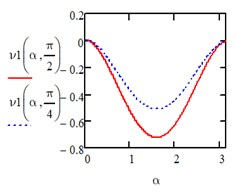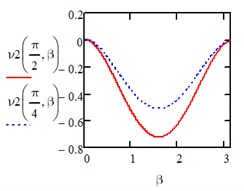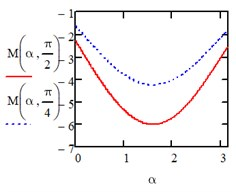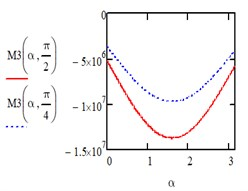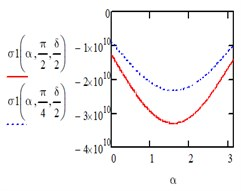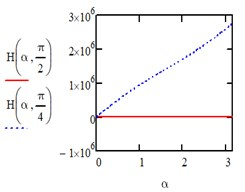Under the action of a uniform load of the entire area we have:

${G}_{nm}=\frac{16q}{{D}_{nm}{\pi }^{2}}\mathrm{s}\mathrm{i}\mathrm{n}\left(\frac{n\pi }{2}\right)\mathrm{s}\mathrm{i}\mathrm{n}\left(\frac{m\pi }{2}\right).$

Fig. 2Computed plot of the evenly distributed load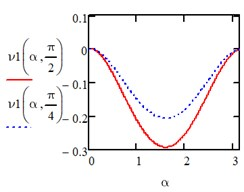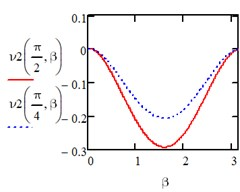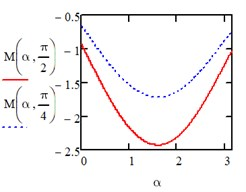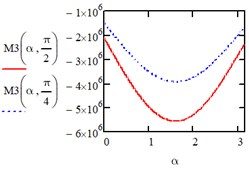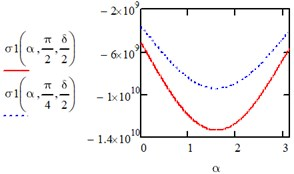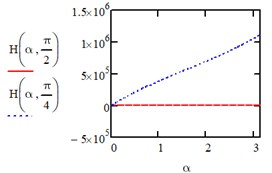Under the action of a load changing along a parabola in the directions of the axis $\alpha$ of the entire area we have:

${G}_{nm}=\frac{32q}{{D}_{nm}^{3}{\pi }^{4}}\left[1-{\left(-1\right)}^{n}\right]\mathrm{s}\mathrm{i}\mathrm{n}\left(\frac{n\pi }{2}\right)\mathrm{s}\mathrm{i}\mathrm{n}\left(\frac{m\pi }{2}\right).$

Fig. 3Computed plot of the changing parabola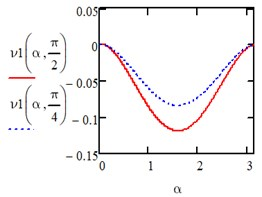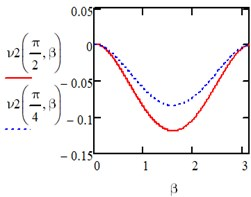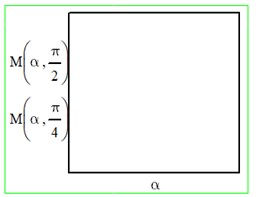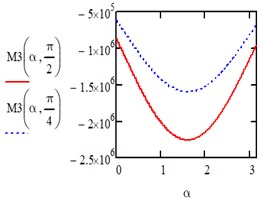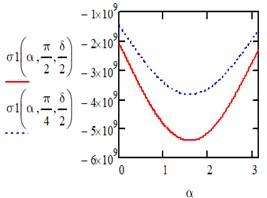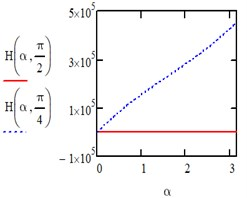When the action is changing along an isosceles triangle in the directions of the axis $\alpha$ of the entire area we have:

${G}_{nm}=\frac{16q}{{D}_{nm}^{2}{\pi }^{3}}\mathrm{s}\mathrm{i}{\mathrm{n}}^{2}\left(\frac{n\pi }{2}\right)\mathrm{s}\mathrm{i}\mathrm{n}\left(\frac{m\pi }{2}\right).$

Fig. 4Computed plot of the isosceles triangle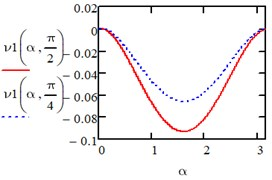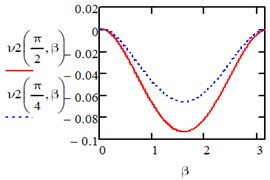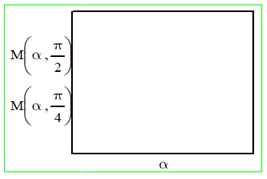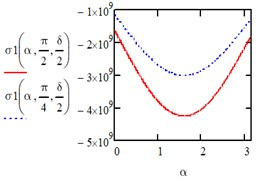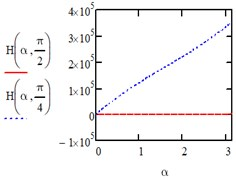## 4. Conclusions

The paper obtained equations of plate bending, internal force factors and stresses, and the following new scientific results:

1) approximate analytical solutions were obtained for the functions of bends, the formulas of internal force factors and stresses given various boundary conditions of the plate and load;

2) the results of a numerical experiment for the plate were obtained given various fastenings of the edges and load;

3) mechanical effects, conditions of fastening along the contour and load were revealed.

#### Cited by

2021 IEEE International Symposium on the Physical and Failure Analysis of Integrated Circuits (IPFA)

20 February 2020
Accepted
26 May 2020
Published
26 November 2020
SUBJECTS
Mathematical models in engineering
Erratum
The institutional affiliation was incorrect for Bidakhmet Zhanar in the paper finally approved (after the acceptance) by the authors. For more information read Editor's Note.
Keywords
mathematical modeling
high-strength
composite material
contour
solving problems